irf

Generate vector error-correction (VEC) model impulse responses

Description

The irf function returns the dynamic response, or the impulse response function (IRF), to a one-standard-deviation shock to each variable in a VEC(p – 1) model. A fully specified vecm model object characterizes the VEC model.

IRFs trace the effects of an innovation shock to one variable on the response of all variables in the system. In contrast, the forecast error variance decomposition (FEVD) provides information about the relative importance of each innovation in affecting all variables in the system. To estimate the FEVD of a VEC model characterized by a vecm model object, see fevd.

example

Response = irf(Mdl) returns the 20-period, orthogonalized IRF of the time series variables that compose the VEC(p – 1) model Mdl characterized by a fully specified vecm model object. irf shocks variables at time 0, and returns the IRF for times 0 through 19.

example

Response = irf(Mdl,Name,Value) uses additional options specified by one or more name-value pair arguments. For example, 'NumObs',10,'Method',"generalized" specifies estimating a generalized IRF for 10 time points starting at time 0, during which irf applies the shock, and ending at period 9.

example

[Response,Lower,Upper] = irf(___) uses any of the input argument combinations in the previous syntaxes and returns lower and upper confidence bounds, for each period and variable in the IRF, of a 95% confidence interval on the true IRF.

• If you specify series of residuals by using the E name-value pair argument, then irf estimates the confidence bounds by bootstrapping the specified residuals.

• Otherwise, irf estimates confidence bounds by conducting Monte Carlo simulation.

If Mdl is a custom vecm model object (an object not returned by estimate or modified after estimation), irf might require a sample size for the simulation SampleSize or presample responses Y0.

Examples

collapse all

Fit a 4-D VEC(2) model to Danish money and income rate series. Then, estimate the orthogonalized IRF from the estimated model.

Load the Danish money and income data set.

The data set includes four time series in the table DataTable. For more details on the data set, enter Description at the command line.

Create a vecm model object that represents a 4-D VEC(2) model with two cointegrating relations. Specify the variable names.

Mdl = vecm(4,2,2);
Mdl.SeriesNames = DataTable.Properties.VariableNames;

Mdl is a vecm model object specifying the structure of a 4-D VEC(2) model; it is a template for estimation.

Fit the VEC(2) model to the data set.

Mdl = estimate(Mdl,DataTable.Series);

Mdl is a fully specified vecm model object representing an estimated 4-D VEC(2) model.

Estimate the orthogonalized IRF from the estimated VEC(2) model.

Response = irf(Mdl);

Response is a 20-by-4-by-4 array representing the IRF of Mdl. Rows correspond to consecutive time points from time 0 to 19, columns correspond to variables receiving a one-standard-deviation innovation shock at time 0, and pages correspond to responses of variables to the variable being shocked. Mdl.SeriesNames specifies the variable order.

Display the IRF of the bond rate (variable 3, IB) when the log of real income (variable 2, Y) is shocked at time 0.

Response(:,2,3)
ans = 20×1

0.0021
0.0057
0.0064
0.0067
0.0064
0.0061
0.0057
0.0056
0.0057
0.0058
⋮

The armairf function plots the IRF of VAR models characterized by AR coefficient matrices. Plot the IRF of a VEC model by:

1. Expressing the VEC(2) model as a VAR(3) model by passing Mdl to varm

2. Passing the VAR model AR coefficients and innovations covariance matrix to armairf

Plot the VEC(2) model IRF for 40 periods.

VARMdl = varm(Mdl);
armairf(VARMdl.AR,[],"InnovCov",VARMdl.Covariance,...
"NumObs",40);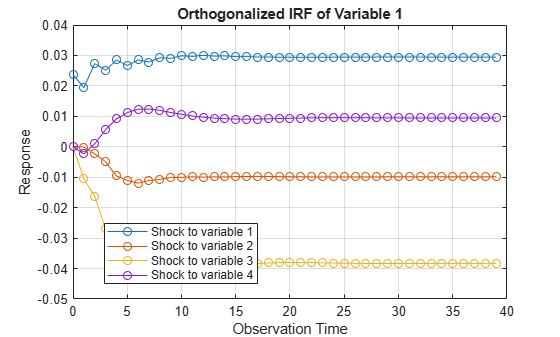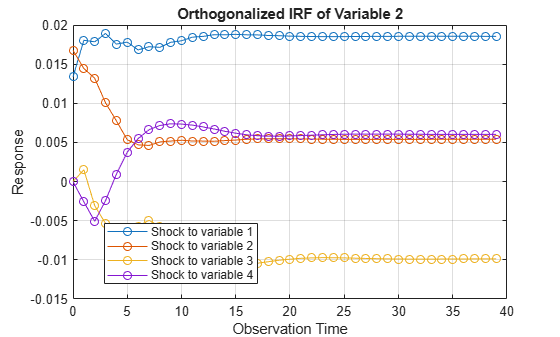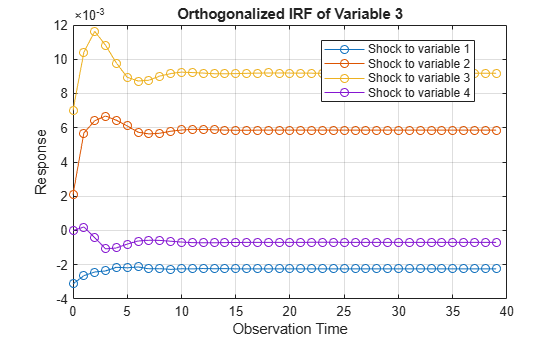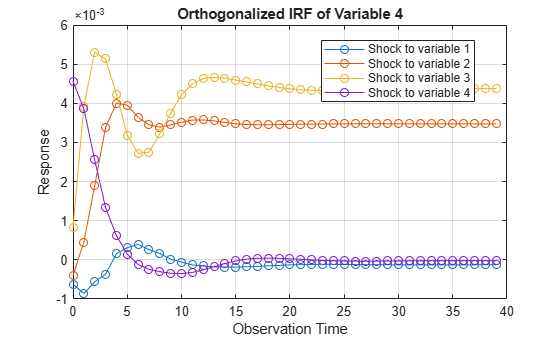Each plot shows the four IRFs of a variable when all other variables are shocked at time 0. Mdl.SeriesNames specifies the variable order.

Consider the 4-D VEC(2) model with two cointegrating relations in Estimate and Plot VEC Model IRF. Estimate the generalized IRF of the system for 50 periods.

Load the Danish money and income data set, then estimate the VEC(2) model.

Mdl = vecm(4,2,2);
Mdl.SeriesNames = DataTable.Properties.VariableNames;
Mdl = estimate(Mdl,DataTable.Series);

Estimate the generalized IRF from the estimated VEC(2) model.

Response = irf(Mdl,"Method","generalized","NumObs",50);

Response is a 50-by-4-by-4 array representing the generalized IRF of Mdl.

Plot the generalized IRF of the bond rate when real income is shocked at time 0.

figure;
plot(0:49,Response(:,2,3))
title("IRF of IB When Y Is Shocked")
xlabel("Observation Time")
ylabel("Response")
grid on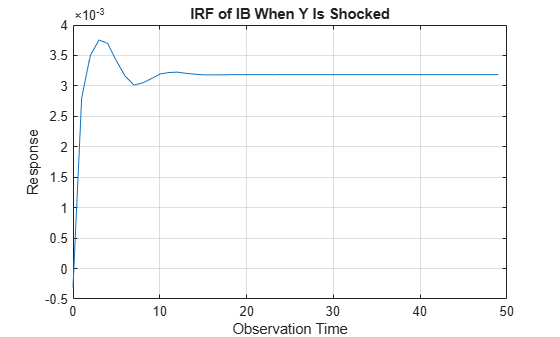When real income is shocked, the bond rate reacts and then settles at approximately 0.0032 after 15 periods.

Consider the 4-D VEC(2) model with two cointegrating relations in Estimate and Plot VEC Model IRF. Estimate and plot its orthogonalized IRF and 95% Monte Carlo confidence intervals on the true IRF.

Load the Danish money and income data set, then estimate the VEC(2) model.

Mdl = vecm(4,2,2);
Mdl.SeriesNames = DataTable.Properties.VariableNames;
Mdl = estimate(Mdl,DataTable.Series);

Estimate the IRF and corresponding 95% Monte Carlo confidence intervals from the estimated VEC(2) model.

rng(1); % For reproducibility
[Response,Lower,Upper] = irf(Mdl);

Response, Lower, and Upper are 20-by-4-by-4 arrays representing the orthogonalized IRF of Mdl and corresponding lower and upper bounds of the confidence intervals. For all arrays, rows correspond to consecutive time points from time 0 to 19, columns correspond to variables receiving a one-standard-deviation innovation shock at time 0, and pages correspond to responses of variables to the variable being shocked. Mdl.SeriesNames specifies the variable order.

Plot the orthogonalized IRF with its confidence bounds of the bond rate when real income is shocked at time 0.

irfshock2resp3 = Response(:,2,3);
IRFCIShock2Resp3 = [Lower(:,2,3) Upper(:,2,3)];

figure;
h1 = plot(0:19,irfshock2resp3);
hold on
h2 = plot(0:19,IRFCIShock2Resp3,'r--');
legend([h1 h2(1)],["IRF" "95% Confidence Interval"])
xlabel("Time Index");
ylabel("Response");
title("IRF of IB When Y Is Shocked");
grid on
hold off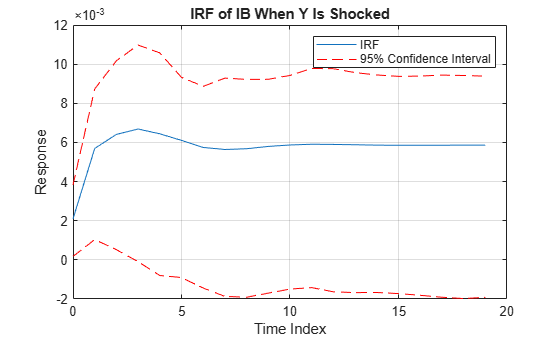When real income is shocked, the bond rate reacts and then settles between –0.002 and 0.0095 with 95% confidence.

Consider the 4-D VEC(2) model with two cointegrating relations in Estimate and Plot VEC Model IRF. Estimate and plot its orthogonalized IRF and 90% bootstrap confidence intervals on the true IRF.

Load the Danish money and income data set, then estimate the VEC(2) model. Return the residuals from model estimation.

Mdl = vecm(4,2,2);
Mdl.SeriesNames = DataTable.Properties.VariableNames;
[Mdl,~,~,E] = estimate(Mdl,DataTable.Series);
T = size(DataTable,1) % Total sample size
T = 55
n = size(E,1)         % Effective sample size
n = 52

E is a 52-by-4 array of residuals. Columns correspond to the variables in Mdl.SeriesNames. The estimate function requires Mdl.P = 3 observations to initialize a VEC(2) model for estimation. Because presample data (Y0) is unspecified, estimate takes the first three observations in the specified response data to initialize the model. Therefore, the resulting effective sample size is TMdl.P = 52, and rows of E correspond to the observation indices 4 through T.

Estimate the orthogonalized IRF and corresponding 90% bootstrap confidence intervals from the estimated VEC(2) model. Draw 500 paths of length n from the series of residuals.

rng(1); % For reproducibility
[Response,Lower,Upper] = irf(Mdl,"E",E,"NumPaths",500,...
"Confidence",0.9);

Plot the orthogonalized IRF with its confidence bounds of the bond rate when real income is shocked at time 0.

irfshock2resp3 = Response(:,2,3);
IRFCIShock2Resp3 = [Lower(:,2,3) Upper(:,2,3)];

figure;
h1 = plot(0:19,irfshock2resp3);
hold on
h2 = plot(0:19,IRFCIShock2Resp3,'r--');
legend([h1 h2(1)],["IRF" "90% Confidence Interval"])
xlabel("Time Index");
ylabel("Response");
title("IRF of IB When Y Is Shocked");
grid on
hold off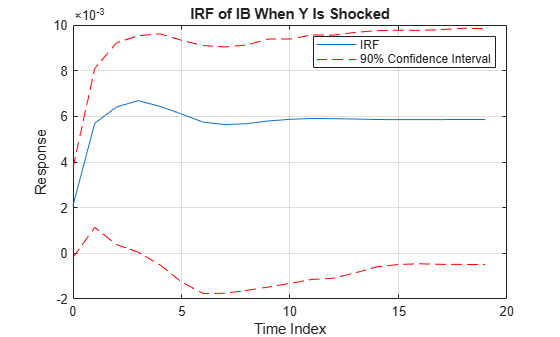When real income is shocked, the bond rate reacts and then settles between approximately 0 and 0.010 with 90% confidence.

Input Arguments

collapse all

VEC model, specified as a vecm model object created by vecm or estimate. Mdl must be fully specified.

Name-Value Arguments

Specify optional comma-separated pairs of Name,Value arguments. Name is the argument name and Value is the corresponding value. Name must appear inside quotes. You can specify several name and value pair arguments in any order as Name1,Value1,...,NameN,ValueN.

Example: 'NumObs',10,'Method',"generalized" specifies estimating a generalized IRF for 10 time points starting at time 0, during which irf applies the shock, and ending at period 9.
Options for All IRFs

collapse all

Number of periods for which irf computes the IRF, specified as the comma-separated pair consisting of 'NumObs' and a positive integer. NumObs specifies the number of observations to include in the IRF (the number of rows in Response).

Example: 'NumObs',10 specifies the inclusion of 10 time points in the IRF starting at time 0, during which irf applies the shock, and ending at period 9.

Data Types: double

IRF computation method, specified as the comma-separated pair consisting of 'Method' and a value in this table.

ValueDescription
"orthogonalized"Compute impulse responses using orthogonalized, one-standard-deviation innovation shocks. irf uses the Cholesky factorization of Mdl.Covariance for orthogonalization.
"generalized"Compute impulse responses using one-standard-deviation innovation shocks.

Example: 'Method',"generalized"

Data Types: string

Options for Confidence Bound Estimation

collapse all

Number of sample paths (trials) to generate, specified as the comma-separated pair consisting of 'NumPaths' and a positive integer.

Example: 'NumPaths',1000 generates 1000 sample paths from which the software derives the confidence bounds.

Data Types: double

Number of observations for the Monte Carlo simulation or bootstrap per sample path, specified as the comma-separated pair consisting of 'SampleSize' and a positive integer.

• If Mdl is an estimated vecm model object (an object returned by estimate and unmodified thereafter), then the default is the sample size of the data to which the model is fit (see summarize).

• If irf estimates confidence bounds by conducting a Monte Carlo simulation (for details, see E), you must specify SampleSize.

• If irf estimates confidence bounds by bootstrapping residuals, the default is the length of the specified series of residuals (size(E,1)).

Example: If you specify 'SampleSize',100 and do not specify the 'E' name-value pair argument, the software estimates confidence bounds from NumPaths random paths of length 100 from Mdl.

Example: If you specify 'SampleSize',100,'E',E, the software resamples, with replacement, 100 observations (rows) from E to form a sample path of innovations to filter through Mdl. The software forms NumPaths random sample paths from which it derives confidence bounds.

Data Types: double

Presample response data that provides initial values for model estimation during the simulation, specified as the comma-separated pair consisting of 'Y0' and a numpreobs-by-numseries numeric matrix.

Rows of Y0 correspond to periods in the presample, and the last row contains the latest presample response. numpreobs is the number of specified presample responses and it must be at least Mdl.P. If numpreobs exceeds Mdl.P, then irf uses only the latest Mdl.P rows.

numseries is the dimensionality of the input VEC model Mdl.NumSeries. Columns must correspond to the response variables in Mdl.SeriesNames.

• If Mdl is an estimated vecm model object (an object returned by estimate and unmodified thereafter), irf sets Y0 to the presample response data used for estimation by default (see 'Y0').

• Otherwise, you must specify Y0.

Data Types: double

Predictor data for estimating the model regression component during the simulation, specified as the comma-separated pair consisting of 'X' and a numeric matrix containing numpreds columns.

numpreds is the number of predictor variables (size(Mdl.Beta,2)).

Rows correspond to observations. X must have at least SampleSize rows. If you supply more rows than necessary, irf uses only the latest SampleSize observations. The last row contains the latest observation.

Columns correspond to individual predictor variables. All predictor variables are present in the regression component of each response equation.

To maintain model consistency when irf estimates the confidence bounds, a good practice is to specify X when Mdl has a regression component. If Mdl is an estimated model, specify the predictor data used during model estimation (see 'X').

By default, irf excludes the regression component from confidence bound estimation, regardless of its presence in Mdl.

Data Types: double

Series of residuals from which to draw bootstrap samples, specified as the comma-separated pair consisting of 'E' and a numeric matrix containing numseries columns. irf assumes that E is free of serial correlation.

Columns contain the residual series corresponding to the response series names in Mdl.SeriesNames.

If Mdl is an estimated vecm model object (an object returned by estimate), you can specify E as the inferred residuals from estimation (see E or infer).

By default, irf derives confidence bounds by conducting a Monte Carlo simulation.

Data Types: double

Confidence level for the confidence bounds, specified as a numeric scalar in the interval [0,1].

For each period, randomly drawn confidence intervals cover the true response 100*Confidence% of the time.

The default value is 0.95, which implies that the confidence bounds represent 95% confidence intervals.

Data Types: double

Johansen form of the VEC(p – 1) model deterministic terms , specified as the comma-separated pair consisting of 'Model' and a value in this table (for variable definitions, see Vector Error-Correction Model).

ValueError-Correction TermDescription
"H2"

AB´yt − 1

No intercepts or trends are present in the cointegrating relations, and no deterministic trends are present in the levels of the data.

Specify this model only when all response series have a mean of zero.

"H1*"

A(B´yt−1+c0)

Intercepts are present in the cointegrating relations, and no deterministic trends are present in the levels of the data.

"H1"

A(B´yt−1+c0)+c1

Intercepts are present in the cointegrating relations, and deterministic linear trends are present in the levels of the data.

"H*"A(B´yt−1+c0+d0t)+c1

Intercepts and linear trends are present in the cointegrating relations, and deterministic linear trends are present in the levels of the data.

"H"A(B´yt−1+c0+d0t)+c1+d1t

Intercepts and linear trends are present in the cointegrating relations, and deterministic quadratic trends are present in the levels of the data.

If quadratic trends are not present in the data, this model can produce good in-sample fits but poor out-of-sample forecasts.

For more details on the Johansen forms, see estimate.

• If Mdl is an estimated vecm model object (an object returned by estimate and unmodified thereafter), the default is the Johansen form used for estimation (see 'Model').

• Otherwise, the default is "H1".

Tip

A best practice is to maintain model consistency during the simulation that estimates confidence bounds. Therefore, if Mdl is an estimated vecm model object (an object returned by estimate and unmodified thereafter), incorporate any constraints imposed during estimation by deferring to the default value of Model.

Example: 'Model',"H1*"

Data Types: string | char

Output Arguments

collapse all

IRF, returned as a numobs-by-numseries-by-numseries numeric array. numobs is the value of NumObs. Columns and pages correspond to the response variables in Mdl.SeriesNames.

Response(t + 1,j,k) is the impulse response of variable k to a one-standard-deviation innovation shock to variable j at time 0, for t = 0, 1, ..., numObs – 1, j = 1,2,...,numseries, and k = 1,2,...,numseries.

Lower confidence bounds, returned as a numobs-by-numseries-by-numseries numeric array. Elements of Lower correspond to elements of Response.

Lower(t + 1,j,k) is the lower bound of the 100*Confidence% percentile interval on the true impulse response of variable k to a one-standard-deviation innovation shock to variable j at time 0.

Upper confidence bounds, returned as a numobs-by-numseries-by-numseries numeric array. Elements of Upper correspond to elements of Response.

Upper(t + 1,j,k) is the upper bound of the 100*Confidence% percentile interval on the true impulse response of variable k to a one-standard-deviation innovation shock to variable j at time 0.

collapse all

Impulse Response Function

An impulse response function (IRF) of a time series model (or dynamic response of the system) measures the changes in the future responses of all variables in the system when a variable is shocked by an impulse. In other words, the IRF at time t is the derivative of the responses at time t with respect to an innovation at time t0 (the time that innovation was shocked), tt0.

Consider a numseries-D VEC(p – 1) model for the multivariate response variable yt. In lag operator notation, the equivalent VAR(p) representation of a VEC(p – 1) model is:

$\Gamma \left(L\right){y}_{t}=c+dt+\beta {x}_{t}+{\epsilon }_{t},$

where $\Gamma \left(L\right)=I-{\Gamma }_{1}L-{\Gamma }_{2}{L}^{2}-...-{\Gamma }_{p}{L}^{p}$ and I is the numseries-by-numseries identity matrix.

In lag operator notation, the infinite lag MA representation of yt is:

$\begin{array}{c}{y}_{t}={\Gamma }^{-1}\left(L\right)\left(c+\beta {x}_{t}+dt\right)+{\Gamma }^{-1}\left(L\right){\epsilon }_{t}\\ =\Omega \left(L\right)\left(c+\beta {x}_{t}+dt\right)+\Omega \left(L\right){\epsilon }_{t}.\end{array}$

The general form of the IRF of yt shocked by an impulse to variable j by one standard deviation of its innovation m periods into the future is:

${\psi }_{j}\left(m\right)={C}_{m}{e}_{j}.$

• ej is a selection vector of length numseries containing a 1 in element j and zeros elsewhere.

• For the orthogonalized IRF, ${C}_{m}={\Omega }_{m}P,$ where P is the lower triangular factor in the Cholesky factorization of Σ, and Ωm is the lag m coefficient of Ω(L).

• For the generalized IRF, ${C}_{m}={\sigma }_{j}^{-1}{\Omega }_{m}\Sigma ,$ where σj is the standard deviation of innovation j.

• The IRF is free of the model constant, regression component, and time trend.

Vector Error-Correction Model

A vector error-correction (VEC) model is a multivariate, stochastic time series model consisting of a system of m = numseries equations of m distinct, differenced response variables. Equations in the system can include an error-correction term, which is a linear function of the responses in levels used to stabilize the system. The cointegrating rank r is the number of cointegrating relations that exist in the system.

Each response equation can include an autoregressive polynomial composed of first differences of the response series (short-run polynomial of degree p – 1), a constant, a time trend, exogenous predictor variables, and a constant and time trend in the error-correction term.

A VEC(p – 1) model in difference-equation notation and in reduced form can be expressed in two ways:

• This equation is the component form of a VEC model, where the cointegration adjustment speeds and cointegration matrix are explicit, whereas the impact matrix is implied.

$\begin{array}{c}\Delta {y}_{t}=A\left(B\prime {y}_{t-1}+{c}_{0}+{d}_{0}t\right)+{c}_{1}+{d}_{1}t+{\Phi }_{1}\Delta {y}_{t-1}+...+{\Phi }_{p-1}\Delta {y}_{t-\left(p-1\right)}+\beta {x}_{t}+{\epsilon }_{t}\\ =c+dt+AB\prime {y}_{t-1}+{\Phi }_{1}\Delta {y}_{t-1}+...+{\Phi }_{p-1}\Delta {y}_{t-\left(p-1\right)}+\beta {x}_{t}+{\epsilon }_{t}.\end{array}$

The cointegrating relations are B'yt – 1 + c0 + d0t and the error-correction term is A(B'yt – 1 + c0 + d0t).

• This equation is the impact form of a VEC model, where the impact matrix is explicit, whereas the cointegration adjustment speeds and cointegration matrix are implied.

$\begin{array}{c}\Delta {y}_{t}=\Pi {y}_{t-1}+A\left({c}_{0}+{d}_{0}t\right)+{c}_{1}+{d}_{1}t+{\Phi }_{1}\Delta {y}_{t-1}+...+{\Phi }_{p-1}\Delta {y}_{t-\left(p-1\right)}+\beta {x}_{t}+{\epsilon }_{t}\\ =c+dt+\Pi {y}_{t-1}+{\Phi }_{1}\Delta {y}_{t-1}+...+{\Phi }_{p-1}\Delta {y}_{t-\left(p-1\right)}+\beta {x}_{t}+{\epsilon }_{t}.\end{array}$

In the equations:

• yt is an m-by-1 vector of values corresponding to m response variables at time t, where t = 1,...,T.

• Δyt = ytyt – 1. The structural coefficient is the identity matrix.

• r is the number of cointegrating relations and, in general, 0 < r < m.

• A is an m-by-r matrix of adjustment speeds.

• B is an m-by-r cointegration matrix.

• Π is an m-by-m impact matrix with a rank of r.

• c0 is an r-by-1 vector of constants (intercepts) in the cointegrating relations.

• d0 is an r-by-1 vector of linear time trends in the cointegrating relations.

• c1 is an m-by-1 vector of constants (deterministic linear trends in yt).

• d1 is an m-by-1 vector of linear time-trend values (deterministic quadratic trends in yt).

• c = Ac0 + c1 and is the overall constant.

• d = Ad0 + d1 and is the overall time-trend coefficient.

• Φj is an m-by-m matrix of short-run coefficients, where j = 1,...,p – 1 and Φp – 1 is not a matrix containing only zeros.

• xt is a k-by-1 vector of values corresponding to k exogenous predictor variables.

• β is an m-by-k matrix of regression coefficients.

• εt is an m-by-1 vector of random Gaussian innovations, each with a mean of 0 and collectively an m-by-m covariance matrix Σ. For ts, εt and εs are independent.

Condensed and in lag operator notation, the system is

$\begin{array}{c}\Phi \left(L\right)\left(1-L\right){y}_{t}=A\left(B\prime {y}_{t-1}+{c}_{0}+{d}_{0}t\right)+{c}_{1}+{d}_{1}t+\beta {x}_{t}+{\epsilon }_{t}\\ =c+dt+AB\prime {y}_{t-1}+\beta {x}_{t}+{\epsilon }_{t}\end{array}$

where $\Phi \left(L\right)=I-{\Phi }_{1}-{\Phi }_{2}-...-{\Phi }_{p-1}$, I is the m-by-m identity matrix, and Lyt = yt – 1.

If m = r, then the VEC model is a stable VAR(p) model in the levels of the responses. If r = 0, then the error-correction term is a matrix of zeros, and the VEC(p – 1) model is a stable VAR(p – 1) model in the first differences of the responses.

Algorithms

• NaN values in Y0, X, and E indicate missing data. irf removes missing data from these arguments by list-wise deletion. Each argument, if a row contains at least one NaN, then irf removes the entire row.

List-wise deletion reduces the sample size, can create irregular time series, and can cause E and X to be unsynchronized.

• If Method is "orthogonalized", then the resulting IRF depends on the order of the variables in the time series model. If Method is "generalized", then the resulting IRF is invariant to the order of the variables. Therefore, the two methods generally produce different results.

• If Mdl.Covariance is a diagonal matrix, then the resulting generalized and orthogonalized IRFs are identical. Otherwise, the resulting generalized and orthogonalized IRFs are identical only when the first variable shocks all variables (that is, all else being the same, both methods yield the same value of Response(:,1,:)).

• The predictor data X represents a single path of exogenous multivariate time series. If you specify X and the VAR model Mdl has a regression component (Mdl.Beta is not an empty array), irf applies the same exogenous data to all paths used for confidence interval estimation.

• irf conducts a simulation to estimate the confidence bounds Lower and Upper.

• If you do not specify residuals E, then irf conducts a Monte Carlo simulation by following this procedure:

1. Simulate NumPaths response paths of length SampleSize from Mdl.

2. Fit NumPaths models that have the same structure as Mdl to the simulated response paths. If Mdl contains a regression component and you specify X, then irf fits the NumPaths models to the simulated response paths and X (the same predictor data for all paths).

3. Estimate NumPaths IRFs from the NumPaths estimated models.

4. For each time point t = 0,…,NumObs, estimate the confidence intervals by computing 1 – Confidence and Confidence quantiles (upper and lower bounds, respectively).

• If you specify residuals E, then irf conducts a nonparametric bootstrap by following this procedure:

1. Resample, with replacement, SampleSize residuals from E. Perform this step NumPaths times to obtain NumPaths paths.

2. Center each path of bootstrapped residuals.

3. Filter each path of centered, bootstrapped residuals through Mdl to obtain NumPaths bootstrapped response paths of length SampleSize.

4. Complete steps 2 through 4 of the Monte Carlo simulation, but replace the simulated response paths with the bootstrapped response paths.

 Hamilton, James D. Time Series Analysis. Princeton, NJ: Princeton University Press, 1994.

 Johansen, S. Likelihood-Based Inference in Cointegrated Vector Autoregressive Models. Oxford: Oxford University Press, 1995.

 Juselius, K. The Cointegrated VAR Model. Oxford: Oxford University Press, 2006.

 Pesaran, H. H., and Y. Shin. "Generalized Impulse Response Analysis in Linear Multivariate Models." Economic Letters. Vol. 58, 1998, pp. 17–29.